The Real Transistor Amplifier Go to Talking Electronics website

STARTING
In this article you are going to learn how to really design a transistor amplifier and forget everything you have been shown and told and learnt.

You are going to learn that you cannot design a a circuit to produce mid-rail biasing as the actual set-point depends on the gain of the transistor and the biasing values are very very critical. Any instructor that says he can set the value does not have a clue about circuits. I have not found a single YouTube video that teaches the correct approach or says anything that you can apply to real-life designing, so you need to start with a completely fresh approach and realise everything is actually done completely different to what you have been taught.

We are going to start with the SELF BIASED TRANSISTOR AMPLIFIER.  Commonly called the COMMON EMITTER AMPLIFIER. (The other circuit is called the H-Bridge configuration)

Here is the circuit. It uses real values because that is the only way to learn. And it does produce a fairly accurate mid-rail biasing.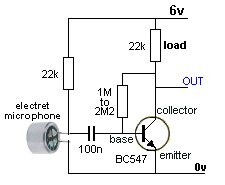Fig1: The REAL Transistor Amplifier

Whistle into the microphone and the circuit will produce a 4 volt waveform. You can change the LOAD resistor from 22k to 47k or down to 10k and the output will alter very little. You can change the base bias resistor from 1M to 2M with very little change in the output waveform.
These changes hardly affect the performance of the circuit because the transistor is creating its own OPERATING POINT. You are not forcing any conditions on the base.

Now we come to the COMMON BASE CIRCUIT described on the web in so many YouTube videos, as the H-Bridge design:Fig2: The H-Bridge Transistor Amplifier

This circuit is actually called the H-BRIDGE Common Emitter Amplifier.
Build the circuit and you will get a shock.  IT DOES NOT WORK.
Unless the lower base bias resistor is between 16k and 19k the circuit does not produce an output.
This is something that not one of the videos tell you. None of the instructors have built the circuit and none of them know what they are talking about.
This circuit is an absolute DISASTER. The base bias resistors are FORCING conditions on the base and if they are not exactly what the transistor accepts, the circuit does not work AT ALL.
You have NO IDEA what the transistor wants or needs or accepts. You have no idea of its value of gain. It is certainly does not like 0.7v base-emitter voltage. It actually wants something like 635mV  But how do you know the exact value????
Everything you have been shown and told is incorrect.
You have to forget all the rubbish you have seen because it will not allow you to design ANYTHING.
You cannot force conditions on a "small-signal stage."  You must allow it to set its own biasing conditions and this circuit DOES NOT DO THAT.
If the lower base-bias resistor is less than 16k there is absolutely no output and if it is above 19k, the output is zero.
At 17.5k, the output is perfect with 4v amplitude.
So, how are you going to get this perfect output.
You have to allow the transistor to set its own conditions of BIASING. These conditions are called QUIESCENT or REST conditions and can only be obtained from the first circuit.
So, you can forget about trying to force this type of circuit into operation and concentrate on the SELF-BIASED design.

Now we come to then next piece of revolutionary understanding.
I can see these circuits working "in my mind."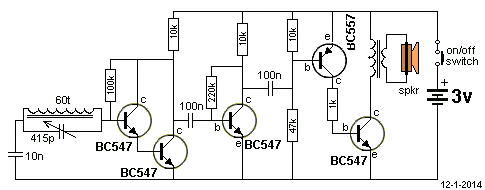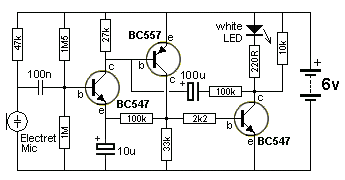It will be years before you can do the same, but we can start with the principles of how a transistor REALLY WORKS.
A transistor is just a VARIABLE RESISTOR - a POTENTIOMETER

Here is the circuit of a REAL TRANSISTOR: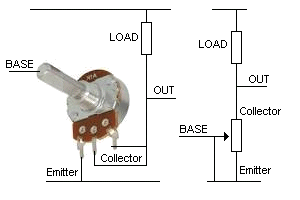Fig3: The Transistor is simply an adjustable resistor

Simply replace the transistor with a POTENTIOMETER. Look at how we have changed the transistor into a "pot."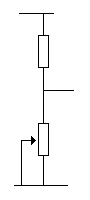Fig4: Two resistors

You now have two resistors in series.
Two RESISTORS IN SERIES is the basis of a VOLTAGE DIVIDER. And that is what each section of a circuit becomes. It becomes a VOLTAGE DIVIDER.
Now I can tell you the most outstanding comment you will ever hear. The potentiometer cannot produce any amplification. No voltage amplification and no current amplification.  And a transistor cannot produce any voltage or current amplification either.  You have  been fooled.
It is the LOAD RESISTOR that provide and produces and assists and is essential in creating these features.
And only when you realise the LOAD RESISTOR creates all the magic, will you understand how a circuit REALLY WORKS.
Just because the transistor increases the base current by 100 or 200, does not make the circuit "move" or "work."
The whole circuit works on the VOLTAGE DIVIDER principle.Fig5: The VOLTAGE DIVIDER

The VOLTAGE DIVIDER is simply two resistor in series. When the lower resistor is a VARIABLE RESISTOR the voltage at the join will INCREASE or DECREASE.

When the resistance of the variable resistor increases, the voltage at the join increases.
That means:  When the resistance of the transistor increases, the output voltage increases.
But you must understand this as:  The top resistor PULLS the voltage high.   The transistor merely ALLOWS the LOAD to pull the output voltage HIGH.
Now, depending on the value of the LOAD RESISTOR, and the ability of the transistor to pull the output voltage LOW, the amplitude of the output is created. The transistor PULLS the output LOW and the LOAD RESISTOR pulls the output voltage HIGH.
But the LOAD RESISTOR can only pull the output voltage HIGH when the transistor stops pulling it LOW.
If the transistor can "pull-down" very strongly, the LOAD resistor can be a small value of resistance and this means the current in the circuit will he high.

HOW DOES THE CIRCUIT WORK?
If the LOAD RESISTOR is a high value of resistance, the transistor will find it very easy to pull the output down to a low value. And this means only a small current will be present.
If the LOAD RESISTOR is a very LOW value of resistance, the transistor will not be able to pull the output down AT ALL (if the transistor is weak).
The input lead of the transistor is the BASE and it needs CURRENT. Current has MUSCLE and it will turn the shaft of the variable resistor to produce a LOW VALUE of resistance.
In actual fact, the transistor increases the "strength" of the input current 100 times (or more) to turn the potentiometer shaft.

THE NEXT POINT
If the value of resistance of the LOAD RESISTOR is HIGH, only a very small current will flow in the voltage divider and only a very small current will be available on the OUTPUT.
If the values of resistance of the LOAD RESISTOR is SMALL, a HIGH current will flow in the voltage divider and a HIGH current will be available on the OUTPUT.
This means the output current will depend on the value of resistance of the LOAD RESISTOR. As long as the transistor can pull the output voltage down to a very small value, the value of current depends only on the  LOAD RESISTOR.
You also have to understand the output voltage depends ONLY
on the LOAD RESISTOR, (when the transistor is able to pull the output voltage LOW).
So, all we have to do make sure the transistor can pull the output voltage LOW and the amplitude and output current capability will depend on the value of the LOAD RESISTOR.

Now, THE NEXT POINT
Here's the next amazing thing you will learn:Fig6: The Self Biased Transistor Amplifier

We will take the Self Biased Transistor Amplifier and see how it works.
When the stage is sitting with no audio input, nothing will be processed.
But a tap on the microphone will reduce is resistance (impedance) and the voltage to the 100n coupling capacitor will fluctuate up and down and when the cycle produces a rising voltage, the first 22k LOAD RESISTOR will do the "pulling up."
Now, what we say is:   22k is entering the transistor amplifier and the transistor reduces this value by 100 to 200 - as this the gain of the transistor - and it becomes 22,000/100 = 220 ohms.
That's right!!   The transistor deals with the input and turns it into a 220 ohm resistor, exactly like fig7: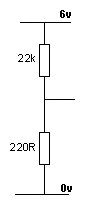Fig7: 22k:220R voltage divider

All you have to do is work out what voltage will appear at the join and you will find it only a very small voltage and that's how the circuit has the potential to produce a very good output waveform. And if I go into further investigation I will get a very accurate outcome without any mathematics or equations or frustration.
I will get a much closer result than any mathematician because I KNOW WHAT I AM DOING.
Technically we have not achieved much in this circuit because the first load resistor is the same value as the second. But we have created an increase in amplitude.  But the main aim for each stage is to create an increase in current. Otherwise the stage is called an INTERFACE STAGE to connect two items with different impedances.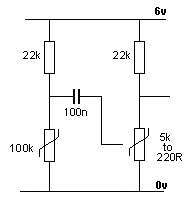Fig8:
The REAL circuit

When designing and diagnosing the Self Biased Stage (with electret microphone), Fig 8 converts all the active components into variable resistors as shown by the symbol that will be new to you..
That's all I see.   The electret microphone can pull the 22k down a small amount and then the capability of the 22k will pass to the transistor via the 100n and get converted to 220R and this value, combined with 22k LOAD resistor will produce a HIGH and LOW output equal to about the 6v supply.Now we come to a 5 Transistor Radio.
We have to convert a 5mV signal with 1microamp capability into a 3v signal with about 50milliamp capability.
The first stage consists of 2 transistors "on top of each other."
This is simply a single transistor with a very high input resistance (impedance), so it does not put a load on the tuned circuit.
Basically it will multiply the 1 microamp by a factor of about 100 and the other transistor will increase this 100 times.  So 1 microamp becomes  100 microamps  x 100 = 10,000 microamps which is 10 milliamps.  But lets reduce this to 1 milliamp. One milliamp flowing through 10k, resistor will produce a voltage drop of 10v. But we only have 3v, so the output will be quite sufficient for the radio.
So, now we have theoretically plenty of current and a high voltage swing.
The next stage simply increases the swing slightly and brings the current up to 1mA.
The final stage consists of two directly-coupled transistors.
This is the job or function result they must perform or carry-out.
The signal into this section has an impedance of about 10k and a current of less than 0.3mA and maybe a voltage swing of 2v.
The stage is required to deliver a current to a load that comprises the primary of speaker transformer and it may be 100 ohms. The maximum current that this load will allow to flow is 3/100 = 30mA.
So, we basically have sufficient amplitude by need a current gain of 30/0.3 = 100.
But the part that is very understood is the IMPEDANCE MATCHING.
The input impedance to the stage is 10k and the output impedance is 100 ohms. This is 10,000/100 = 100:1
In simple terms this means that if you try to drive a signal with 10k impedance into 100 ohms, you will only be able to get 1% success.
This circuit is called an IMPEDANCE MATCHING circuit and does the opposite job to a normal amplifier circuit.
A normal circuit matches a 10k input to a 1k output. One of the best ways to get impedance matching is DIRECT COUPLING and the two transistors are directly coupled to each other.
There are very few ways to this and a PNP NPN pair is the best.
The two transistors do these two things: They match the high impedance input to a low impedance output.
They increase 0.3mA current capability to about 30mAThe two transistors are replaced with variable resistors and the top variable resistor will be reduced in resistance by the signal through the 100n capacitor.
It will then turn ON the lower transistor with a large current because the two are connected with a 1k resistor. This large current will allow a high current to flow in the primary of the speaker transformer to produce a loud output. The 1k resistor tells you this will be possible.

CONSTANT VOLUME HEARING AID

We mentioned at the beginning that you cannot force conditions on the base of a transistor.
Here is a circuit that automatically adjusts the voltage on the base of the first transistor and this effectively changes the gain of the transistor to produce a constant volume, no matter how loud or quiet the surroundings.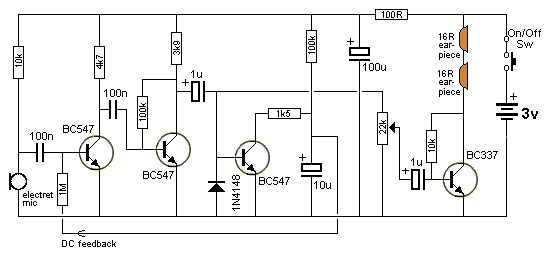We have covered all the stages except the third transistor.
When  the circuit is quiet the transistor is not turned on at all and the 10u is charged via the 100k resistor. This put a voltage on the feedback line to the base of the first transistor.
The circuit is now very sensitive and will pick up the slightest sound.
This faint sound will pass through the first two stages and into the base of the third transistor.

ANOTHER EXAMPLE
If you don't follow my reasoning on SEEING A TRANSISTOR AS A VARIABLE RESISTOR, how are you going to design this type of circuit:
The transistor receives Infrared light from a LED and the resistance between the collector and emitter leads reduces to a point where pin 5 of the microcontroller sees a low of less than 1.3v
The characteristics of the IR transistor are:  1M in darkness and 10k or less when detecting IR light.
But this circuit not very sensitive with the 10k collector resistor and the IR LED has to be very close to the IR transistor for the microcontroller to register a LOW. .
How do you improve the sensitivity?
The IR transistor does not have a base lead, so all your theory about biasing the base of a transistor is worthless. It does not apply.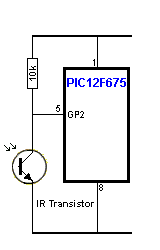That's why you have to see the IR transistor as a variable resistor ,, ,  as shown in the following diagram. When the potentiometer shaft is rotated to full resistance, a voltage-divider is set-up between the pot and 10k load resistor. . . . . . . 10k is in series with 1Meg ohms. You need to understand voltage dividers and very little voltage will be "dropped" across the 10k.  If the supply is 5v, pin5 of the micro will see 4.95v
The IR transistor can on be turned on by the IR light and when it is turned ON by very bright light, the resistance across the collector-emitter leads is 10k.
The voltage divider becomes 10k in series with 10k and the voltage at the join is 2.5v.   This is not LOW enough for the microcontroller to register a LOW and the circuit DOES NOT WORK.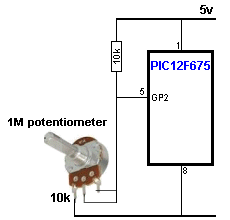The solution is to increase the LOAD resistor to 100k.  The voltage at the join of the resistors becomes 100k:10k  and to keep things simple, the voltage across the 100k is 4.5v and the input of the microcontroller sees 0.5v. This is low enough for the micro to register a LOW.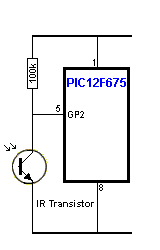This is the IR transistor and 100k LOAD: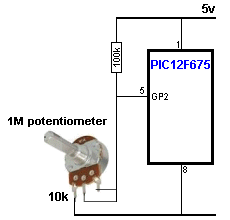Here's the way to look at the circuit. The IR transistor can only pass a certain amount of current.
If the 10k LOAD resistor is connected across the power rails, it will pass twice the current. But if the LOAD resistor is connected to a voltage that is HALF the 5v supply, it will take half the current. This current is the same as the capability of the IR transistor and so, when they are connected in series, they drop the same voltage across each component. That's why the lowest voltage in the first circuit is 50% of rail voltage or 2.5v.
If the IR transistor tries to "pull down" the 10k LOAD resistor, it will want more current from the circuit and the IR transistor cannot pass this extra current and so the two remain at 50%.

If the 10k LOAD is replaced by 100k LOAD, the current through the 100k when connected across the power rails is a lot less than the capability of the IR transistor and so the transistor will be able to "pull down" the 100k until the current wanted by the 100k is equal to the capability of the IR transistor. The transistor is capable of "pulling it down"  to almost the zero volt rail and we are not going into the exact value but it is low enough for the microcontroller to detect a LOW.

This discussion has shown how I "see" circuits working in my mind.

A lot more enlightening discussions and concepts can be found HERE.
https://www.talkingelectronics.com/projects/TheTransistorAmplifier/TheTrAmp/TheTrAmpP1.html

More on the Transistor Amplifier can be found HERE.
https://www.talkingelectronics.com/projects/TheTransistorAmplifier/TheTransistorAmplifier-P1.html

100 Transistor Circuits can be found HERE.
https://www.talkingelectronics.com/projects/200TrCcts/200TrCcts.html

Another 100 Transistor Circuits can be found HERE.
https://www.talkingelectronics.com/projects/200TrCcts/101-200TrCcts.html

After you read and study and understand what I have produced, you will be able to design many types of circuits.  These are called  BUILDING BLOCKS and by connecting blocks together you create circuits that do magical things.

Contact Colin Mitchell if you want any help

Go to Talking Electronics website

2/10/2023